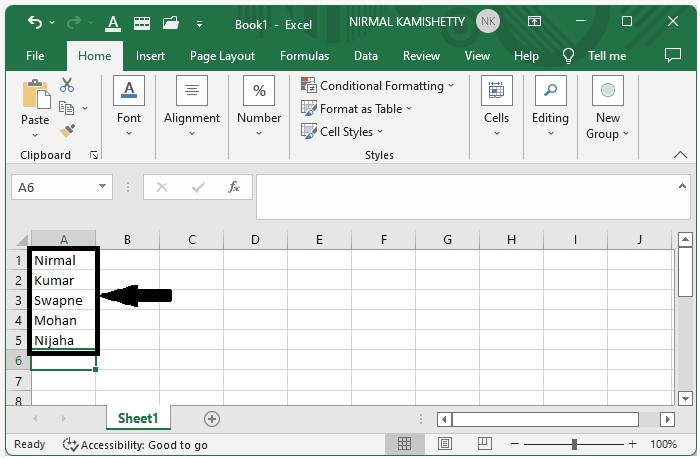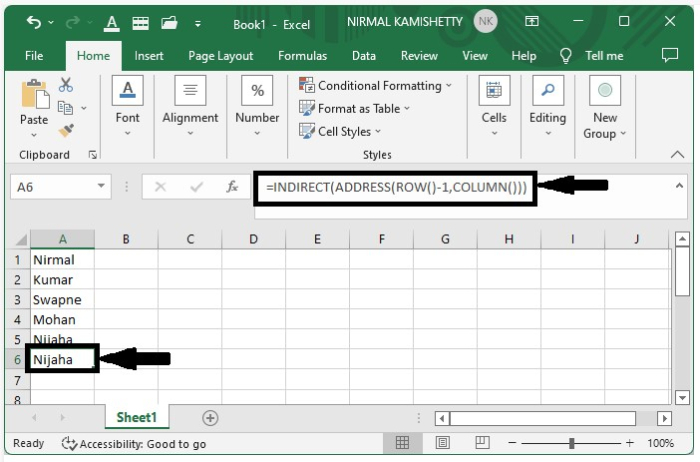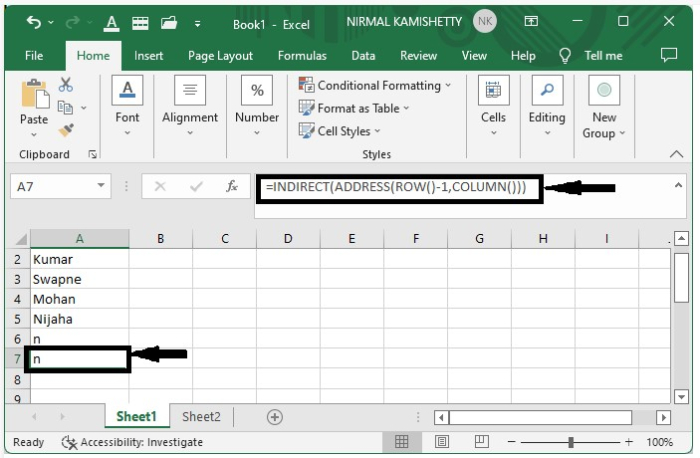# How to Always Get the Value from the Above Cell when Inserting or Deleting a Row in Excel?

When we get the value of the cell from the cell that is present above the selected cell using some formulas, we know that the data is collected from the address mentioned in the formula, but in this case, if we insert a new row into the cell, then all the addresses of the cells will change automatically. There is also a simple process that does not cause the same problem.

This tutorial will help you understand how you can always get the above from the above cell, even when we delete or insert a row in the Excel sheet. This problem will arise in both cases, whether we add the new row or delete the existing one.

## Always Get the Value from the Above Cell when Inserting or Deleting a Row

Here we will use the combination of INDIRECT and ADDRESS formulas to complete the task. Let us see a simple process to understand how we can always get the value from the above cell when inserting or deleting a row.

Step 1

Consider that we have an excel sheet that contains data similar to the data shown in the image below.Now, select the cell that is the last one. In this process, we use the formula as =INDIRECT(ADDRESS(ROW()-1,COLUMN())). This formula does not work based on the address of the cell as all the other formulas do; this formula works based on the current cell.

Now click on the cell and enter the formula as =INDIRECT(ADDRESS(ROW()-1,COLUMN())) in the formula box and press Enter to get the same result as the above cell.Step 2

Now to verify the result, we need to add the new row. To add the new row, right-click on the cell and click on "Insert," then select "Shift cells down" to get the result as shown in the below figure.## Conclusion

In this tutorial, we used a simple example to demonstrate how you can always get the value from the above cell when deleting or inserting rows in Excel to highlight a particular set of data.

Updated on: 09-Jan-2023

380 Views